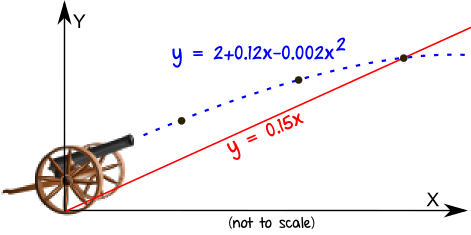## Wave Function

New Topic: Wave Function

Homework: Wave Function Set1

Due: Thursday 12th February

🙂

## The CircleNew Topic: The Circle, MiA Unit 2 Ch4

By the end of this topic you will:

• know that the equation of the circle centre (a,b) and radius r is (x – a)² + (y – b)² = r²

• know that x² + y² + 2gx + 2fy + c = 0 represents a circle centre (–g, –f) and
radius r = √(g² + f² – c), provided g² + f² – c > 0

• be able to determine the equation of a circle

• be able to solve problems with the intersection of a line and a circle,
and a tangent to a circle

• be able to determine whether two circles touch each other

Homework: Circles Set1

Due: Tuesday 13th January

🙂

## Compound Angle FormulaeNew Topic: Compound Angle Formulae, MiA Unit 2 Ch3

By the end of this topic you will be able to:

• know and apply the addition formulae:
sin(A+B) = sinAcosB + cosAsinB
sin(A–B)  = sinAcosB – cosAsinB
cos(A+B) = cosAcosB – sinAsinB
cos(A–B) = cosAcosB + sinAsinB

• know and apply the double angle formulae:
sin2A  = 2sinAcosA
cos2A = cos2A – sin2A = 2cos2A – 1

• apply trigonometric formulae in solution of geometric problems

• solve trigonometric equations using compound angle formulae

🙂

## IntegrationNew Topic: Integration, MiA Unit 2 Ch2

By the end of this topic you will be able to:

• know meaning of terms; integral, integrate, constant of integration,

definite integral, limits of integration, indefinite integral, area under curve

• calculate indefinite integrals

• evaluate definite integrals

• determine area between curve y = f(x), x-axis and lines x = a and x = b

• determine area bounded between two curves

• solve differential equations

Homework: Integration Set1

🙂New Topic: Quadratic Theory, MiA Unit 2 Ch1.2

By the end of this topic you will be able to:
• use the quadratic formula to calculate roots
• know that discriminant of ax² + bx + c = 0 is b² – 4ac
• use discriminant to determine nature of roots of a quadratic
• use discriminant to find condition that the roots of a quadratic are real, equal or unequal
• know condition for tangency; intersection of line and parabola (lines and curves)
• determine a quadratic equation given roots

🙂

## PolynomialsNew Topic: Polynomials, MiA Unit 2 Ch1.1

By the end of this topic you will be able to:
• use the Remainder Theorem to find remainder when dividing by x – h
• determine the roots of a polynomial equation
• use the Factor Theorem to determine the factors of a polynomial
f(x) = (2x – 1)(3x + 2)(2x – 5)

Homework: Polynomials Set1

🙂

## SequencesNew Topic: Sequences, MiA Higher Ch4

By the end of this topic you will:

• know the terms: sequence, nth term, limit as n tends to

• use un notation for the nth term of a sequence

• define and interpret recurrence relations of the form un+1 = mun + c

• know condition for limit of sequence from recurrence relation to exist

• find (where possible) and interpret the limit of a sequence resulting from a recurrence relation in a mathematical model

Homework:

🙂

## DifferentiationNew Topic: Differentiation, MiA Higher Ch3

By the end of this topic you will:
• be familiar with the terminology associated with differentiation
• understand the principles of differentiation
• be able to derive the gradient formula of a given function
• be able to calculate the gradient of a tangent to a curve
• be able to use differentiation to solve optimisation problems

Homework: Differentiation 1 Set1

Due: Thursday 30th October

🙂